# NHÀ khoa học nguyễn văN ĐẠo với lý thuyết dao đỘng và chuyểN ĐỘng hỗN ĐỘN

tải về 3.08 Mb.
 trang 10/11 Chuyển đổi dữ liệu 15.11.2017 Kích 3.08 Mb.
 Table 12. Feigenbaum’s constant in the Poincare’ map Liapunov exponent. To determine the chaotic motion in the system described by (1) it is necessary to calculate the largest Lyapunov exponent. If d0 is a measure of the initial distance between the two starting points, at a small but later time the distance is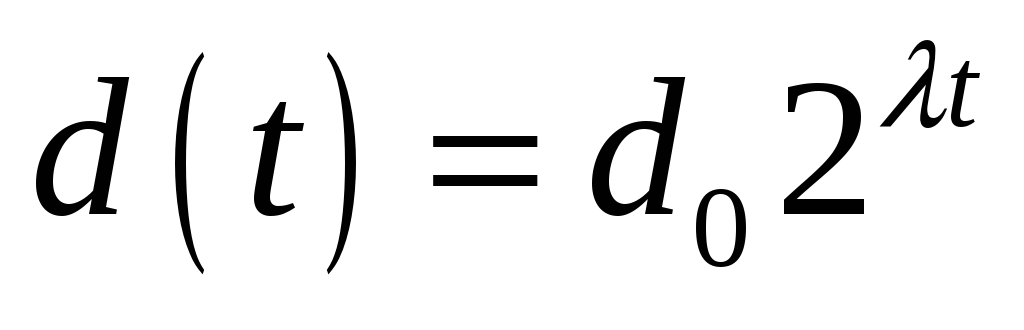The divergence of chaotic orbits can only be locally exponential, since if the system is bounded, as most physical experiments are, d(t) cannot go to infinity. Thus, to define a measure of this divergence of orbits, we must average the exponential growth at many points along a trajectory. One begins with a reference trajectory and a point on a nearby trajectory and measures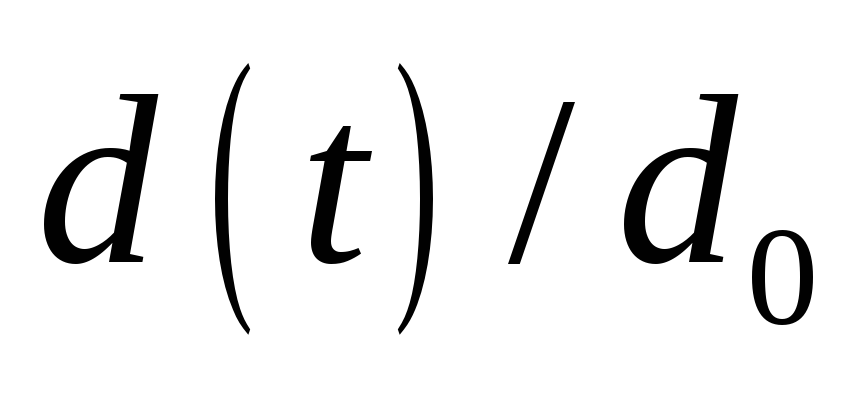. When d(t) becomes too large (i.e, the growth departs from exponential behavior), one looks for a new “nearby” trajectory and defines a new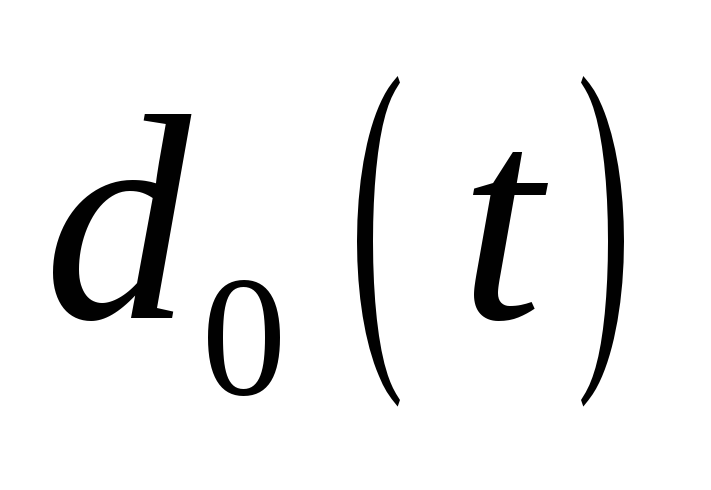. One can define the first Lyapunov exponent by the expression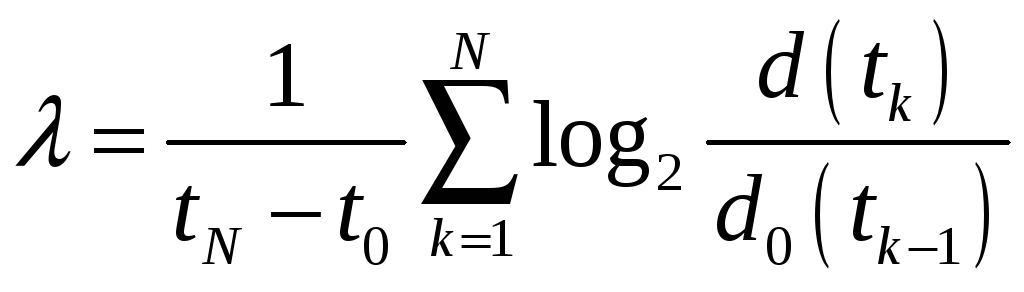the motion is chaotic if the corresponding largest Lyapunov exponent is positive. For this calculation (), in the case of concrete case of Chaotic motion in (2.2), we represent the equation (1) in the form :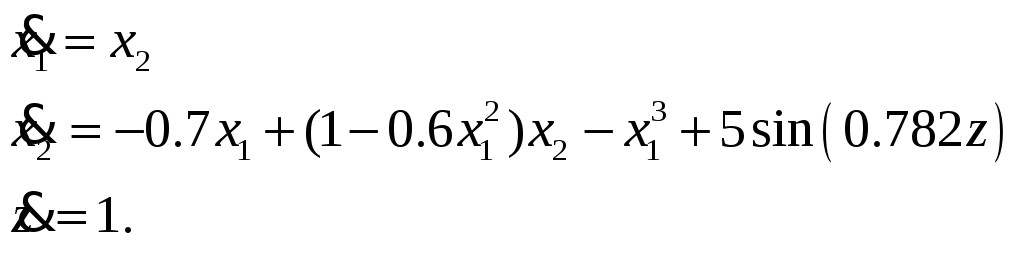(9) Let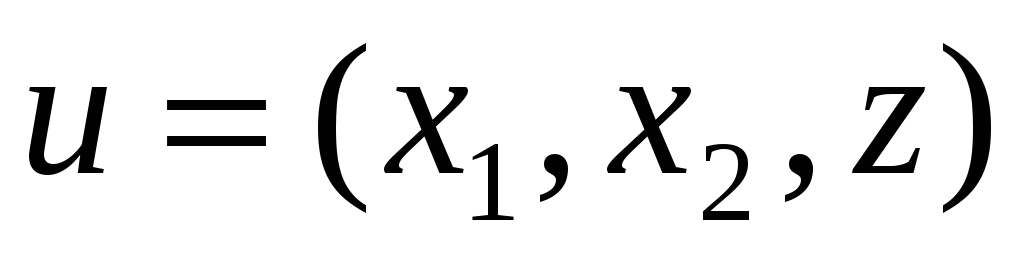is a three dimension vector and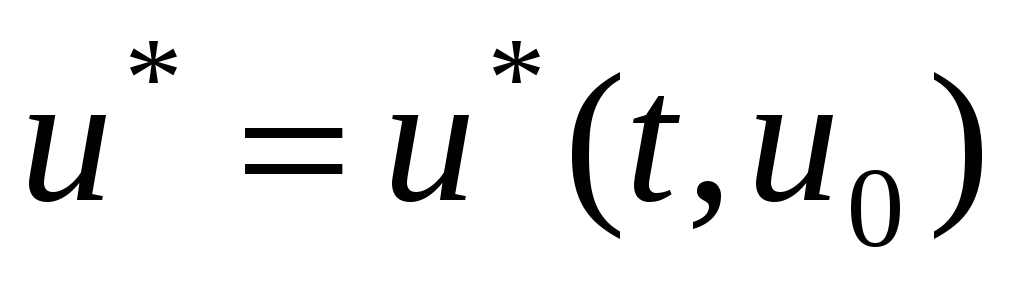is a reference trajectory of the system (3), where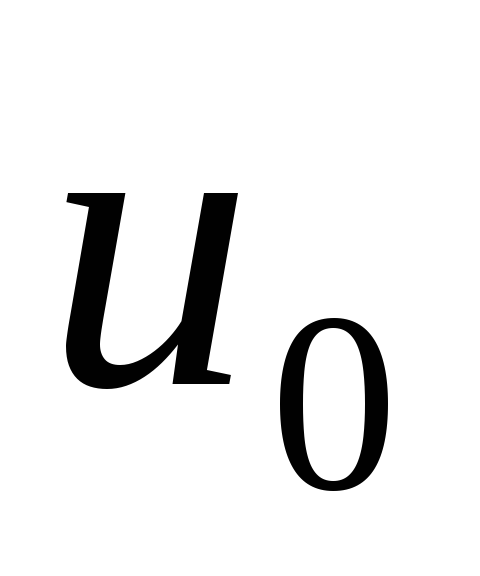is the initial condition. The variational equation corresponding to this reference trajectory is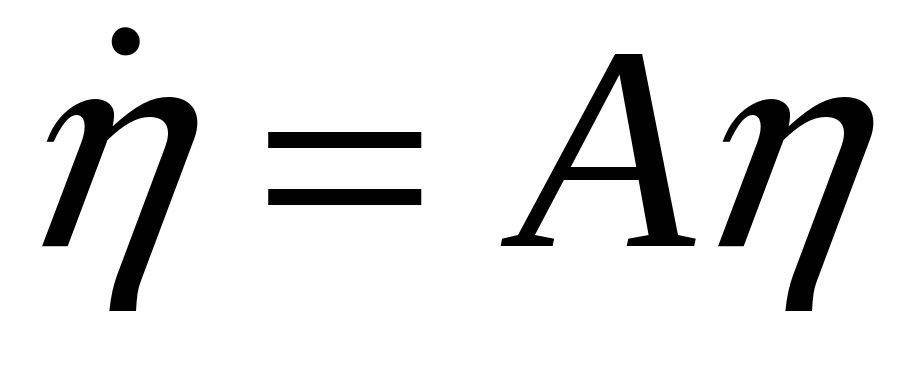, where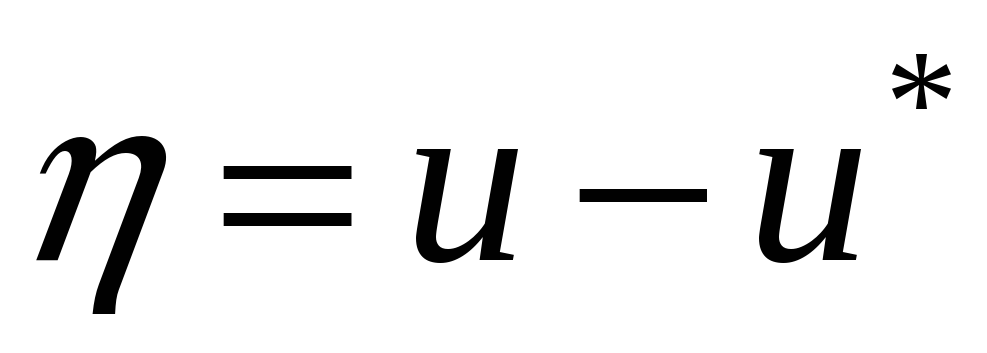and the matrixdepends on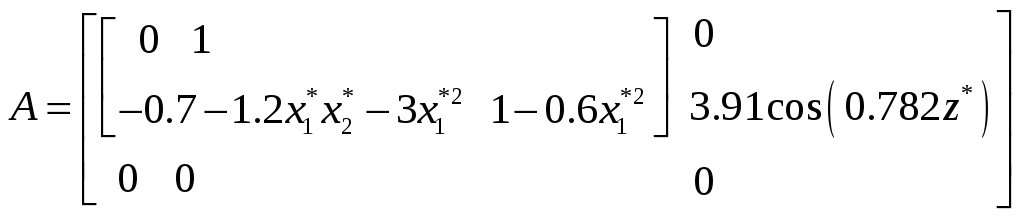. (10) If this initial condition is chosen at random, then it is likely to have a component that lies in the direction of the largest positive eigenvalue of

Cơ sở dữ liệu được bảo vệ bởi bản quyền ©hocday.com 2019
được sử dụng cho việc quản lý

Quê hương